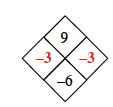### Home > AC > Chapter 1 > Lesson 1.2.2 > Problem1-57

1-57.

Copy and complete each of the Diamond Problems below. The pattern used in the Diamond Problems is shown below.1.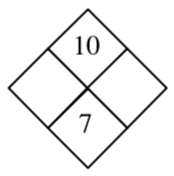What are the factors of $10$?

$1$ and $10$
$2$ and $5$

Which two add to $7$?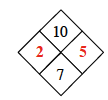1.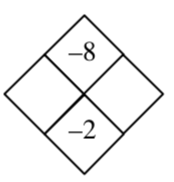Find the factors of $−8$.

The larger factor will be the negative one.

1.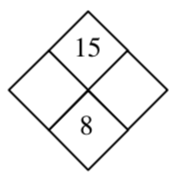Follow the same steps taken in (a).

1.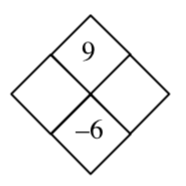Remember that when two negative numbers are multiplied the answer is positive, and when they are added together the answer is negative.